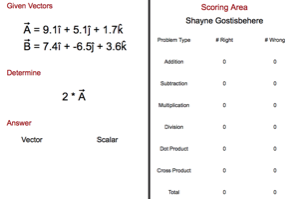Information About The Program
3d Vector Challenge (Game)This challenge is designed to have students practice their three dimensional vector math skills. Students will be asked to add and subtract vectors, multiply and diving vectors by a number, and use the vector dot and cross products.
Below are any Resources that go with this program